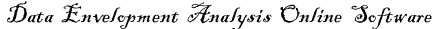# What is DEA?

Data Envelopment Analysis (DEA) is a Linear Programming methodology to measure the relative performance and efficiency of multiple Decision Making Units (DMUs) when the production process presents a difficult structure of multiple inputs and outputs.

Some of the benefits of DEA are:

• no need to explicitly specify a mathematical form for the production function
• proven to be useful in uncovering relationships that remain hidden for other methodologies
• capable of handling multiple inputs and outputs
• capable of being used with any input-output measurement
• the sources of inefficiency can be analysed and quantified for every evaluated unit

In the DEA methodology, formerly developed by Charnes, Cooper and Rhodes (1978) (CCR), efficiency is defined as a weighted sum of outputs to a weighted sum of inputs, where the weights structure is calculated by means of mathematical programming and constant returns to scale (CRS) are assumed. In 1984, Banker, Charnes and Cooper developed a model (BCC) with variable returns to scale (VRS).